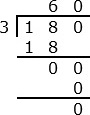# Division With Trailing Zeros Problem Type 2 Online Quiz

Following quiz provides Multiple Choice Questions (MCQs) related to Division With Trailing Zeros: Problem Type 2. You will have to read all the given answers and click over the correct answer. If you are not sure about the answer then you can check the answer using Show Answer button. You can use Next Quiz button to check new set of questions in the quiz.Q 1 - Divide 1600 ÷ 40

A - 20

B - 30

C - 40

D - 50

### Explanation

Step 1:

1600 ÷ 40 = 160 ÷ 4

Step 2: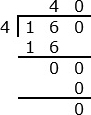Q 2 - Divide 1500 ÷ 30

A - 30

B - 35

C - 40

D - 50

### Explanation

Step 1:

1500 ÷ 30 = 150 ÷ 3

Step 2: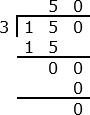Q 3 - Divide 1800 ÷ 90

A - 20

B - 30

C - 40

D - 50

### Explanation

Step 1:

1800 ÷ 90 = 180 ÷ 9

Step 2: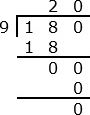Q 4 - Divide 1800 ÷ 60

A - 20

B - 30

C - 40

D - 50

### Explanation

Step 1:

1800 ÷ 60 = 180 ÷ 6

Step 2: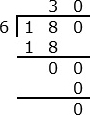Q 5 - Divide 1400 ÷ 70

A - 20

B - 30

C - 40

D - 50

### Explanation

Step 1:

1400 ÷ 70 = 140 ÷ 7

Step 2: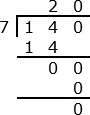Q 6 - Divide 1200 ÷ 60

A - 10

B - 20

C - 30

D - 40

### Explanation

Step 1:

1200 ÷ 60 = 120 ÷ 6

Step 2: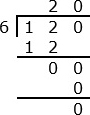Q 7 - Divide 1800 ÷ 50

A - 32

B - 35

C - 36

D - 38

### Explanation

Step 1:

1800 ÷ 50 = 180 ÷ 5

Step 2: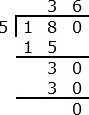Q 8 - Divide 1700 ÷ 50

A - 34

B - 35

C - 36

D - 38

### Explanation

Step 1:

1700 ÷ 50 = 170 ÷ 5

Step 2: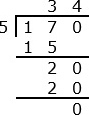Q 9 - Divide 1900 ÷ 50

A - 34

B - 35

C - 36

D - 38

### Explanation

Step 1:

1900 ÷ 50 = 190 ÷ 5

Step 2: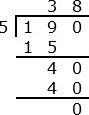Q 10 - Divide 1800 ÷ 30

A - 50

B - 60

C - 70

D - 80